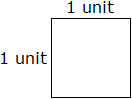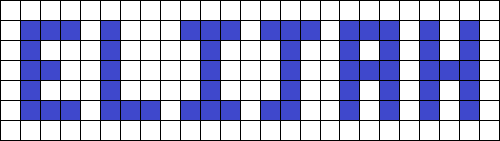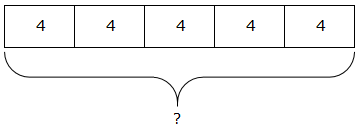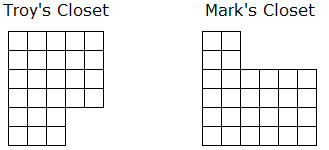Word Problem
Area
What is the product of
Multiplication
100

Julie bought 5 bags of apple slices. There are 10 apple slices in each bag. How many apple slices did Julie buy in all?

50

100

What is the area of the shaded rectangle?10 square centimeters

100

What is the product of 6x6

36

100

Use the model to write a multiplication equation.8x3=24

200

Samson has 5 dog bones in each of the 4 rooms in the house. How many dog bones does Samson have in all?

20

200

What is the area of the shaded rectangle above?9 square centimeters

200

What is the product of 5x12

60

200

Write a multiplication same as this addition expression?

9 + 9 + 9 + 9

4 × 9

300

Mary bought 4 packages of 9 cupcakes. How many cupcakes did Mary buy in all?

27

300

What is the area of this square?1 square units

300

What is the product of 7x4 by using the distributive property?

28

300

Write a equation for the number line show?6 × 3 =

400

There are an unknown number of cats in Tom's barn.

There are 7 whiskers on each cat and 56 whiskers in all.

This story can be written as the number sentence below.

? × 7 = 56

Use the number sentence to find how many cats there are in Tom's barn

8

400

Elijah shaded squares in the grid to make a design that spells his name. Each square in the grid represents 1 square centimeter.

The area of the letter "H" is less than the area of the letter "___"

The area of the letter "J" is greater than the area of the letter "___"The area of the letter "H" is less than the area of the letter "A"

The area of the letter "J" is greater than the area of the letter "L"

400

What is the product of 8x6 by using the distributive property?

48

400Complete the statement.

The number of student desks in Mrs. Young's classroom can be found by using the expression-

___  × ___=____.

The number of student desks in Mrs. Young's classroom can be found by using the expression

5  × 4=20

500If Betty puts the same number of flowers into each vase, which number sentence shows how many flowers will go into each vase?

3 × 3 = 9

500

Robert wants to put carpet squares on the floor of each of his son's closets. Each square in the figures represents 1 square foot.Troy's closet has an area of _____ square feet.

Mark's closet has an area of ______  square feet.

Who has the larger closet?

Troy's 26 square feet

Mark's 28 square feet

Mark has the largest closet.

500

What is the product of 9x7 by using the distributive property?

63

500

Write a multiplication equation and solve.3 × 7=21

Click to zoom